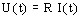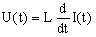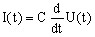# Passive components: resistor, inductor, capacitor

## Introduction

The passive components are of three types:

• the resistor
• the coil
• the capacitor

## Utilization

The passive components are used in the Steady State AC Magnetic and the Magnetic Transient applications.

## Characteristic

The current-voltage characteristic for each of these different components is mentioned again in the table below.

Component

Current-voltage

characteristic

Characteristic quantity Initial value
resistorR is the value of the resistance in (in Ω )
coilL is the value of the coil inductance (in H)

Initial current

I (t = 0)

capacitorC is the value of the capacitance (in F)

Initial voltage

U (t = 0)

where:

• U(t) is the voltage at the terminals of the component (in V)
• I(t) is the current flowing through the component (in A)